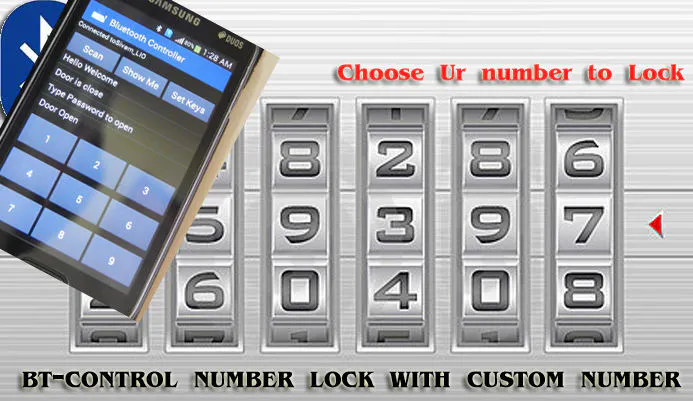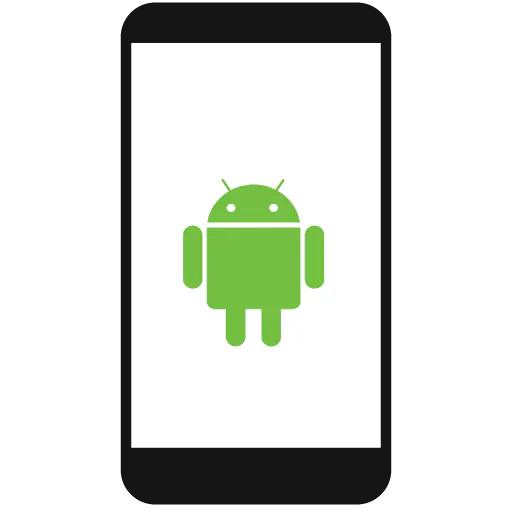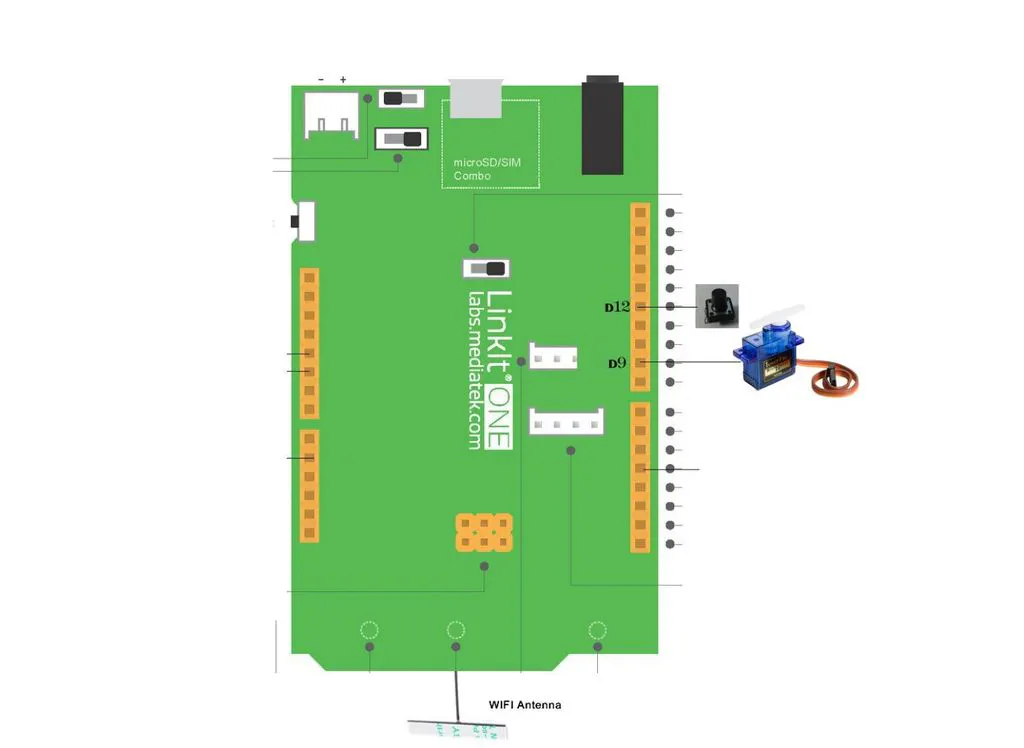# Smart BT Lock with Custom Number

High security cheap number lock. No one can able to see where the lock from outside the door. Use change password when ever they want.## Things used in this project

### Hardware componentsArduino UNO & Genuino UNO
×1SparkFun Pushbutton switch 12mm
×1Android device
×1
 servo motor
×1
 Door Lock
×1

### Software apps and online services

 Bluetooth Controller App

### Hand tools and fabrication machinesSoldering iron (generic)

## Schematics

### Diagram## Code

### BTlocker.ino

Arduino
```#include <LBT.h>
#include <LBTServer.h>
#include <LEEPROM.h>
#include <Servo.h>

Servo myservo;

int resultvalue;
int checktime=0;

String msg;

const int buttonPin = 12;
const int ledPin =  13;

boolean firstconn;
boolean changepass;
int Doorstatus;

void setup() {
if(!LBTServer.begin((uint8_t*)"Sivam_LIO"))
{
return;
}
//  writepass("123456");
//  Serial.begin(9600);
pinMode(ledPin, OUTPUT);
pinMode(buttonPin, INPUT);
firstconn=false;
changepass=false;
myservo.attach(9);
delay(100);
}

void servocommand()
{
if (Doorstatus==0)
{
myservo.write(0);
delay(100);
}
else
{
myservo.write(180);
delay(100);
}
}

void loop() {
uint8_t buf;
if(LBTServer.connected())
{
if (firstconn==false)
{
firstconn=true;
delay(200);
LBTServer.write("Hello Welcome");
delay(100);
if (Doorstatus==0)
{
LBTServer.write("Door is close");
delay(100);
delay(100);
}
else
{
LBTServer.write("Door is open");
delay(100);
delay(100);
}
}
if (digitalRead(buttonPin) == HIGH && Doorstatus==0 && changepass==false)
{
changepass=true;
delay(100);
}
else if (digitalRead(buttonPin) == LOW && changepass==true)
{
changepass=false;
delay(100);
}
while(true)
{
break;
checktime=0;
//     LBTServer.write(msg);
{
if (changepass==false)
{
{
if (Doorstatus==0)
{
Doorstatus=1;
servocommand();
LBTServer.write("Door Open");
}
else
{
Doorstatus=0;
servocommand();
LBTServer.write("Door Close");
}
}
else
{
}
}
else
{
delay(100);
delay(100);
}
}
}
delay(100);

{
checktime=checktime+1;
if (checktime>5)
{
changepass=false;
checktime=0;
LBTServer.write("Time out. Try again");
delay(100);
}
}

}
else
{
firstconn=false;
changepass=false;
LBTServer.accept(5);
}

//  if (digitalRead(buttonPin) == HIGH) {
//    Serial.println(2);
//    digitalWrite(ledPin, HIGH);
//  }
//  else {
//    Serial.println(3);
//    digitalWrite(ledPin, LOW);
//  }

delay(100);
}

{
String mempass;
int memval;
mempass="";
for(int i=0;i<6;i++)
{
if (memval>9)
{
memval=0;
}
mempass=mempass + memval;
}
return mempass;
}
void writepass(String passw)
{
String mempass;
int memval;
for(int i=0;i<6;i++)
{
mempass=passw.substring(i,i+1);
memval=mempass.toInt();
}
}
```

### BTlockerWA.ino

Arduino
```#include <LBT.h>
#include <LBTServer.h>
#include <LEEPROM.h>
//#include <Servo.h>

//Servo myservo;

int resultvalue;
int checktime=0;

String msg;

const int buttonPin = 12;x
//const int ledPin =  13;
const int ctrlpin =  10;

boolean firstconn;
boolean changepass;
int Doorstatus;

void setup() {
if(!LBTServer.begin((uint8_t*)"Sivam_LIO"))
{
return;
}
//  writepass("123456");
//  Serial.begin(9600);
pinMode(ctrlpin, OUTPUT);
pinMode(buttonPin, INPUT);
firstconn=false;
changepass=false;
//  myservo.attach(9);
delay(100);
}

void servocommand()
{
if (Doorstatus==0)
{
digitalWrite(ctrlpin, LOW);
//    myservo.write(0);
//    delay(100);
}
else
{
digitalWrite(ctrlpin, HIGH);
//    myservo.write(180);
//    delay(100);
}
}

void loop() {
uint8_t buf;
if(LBTServer.connected())
{
if (firstconn==false)
{
firstconn=true;
delay(200);
LBTServer.write("Hello Welcome");
delay(100);
if (Doorstatus==0)
{
LBTServer.write("Door is close");
delay(100);
delay(100);
}
else
{
LBTServer.write("Door is open");
delay(100);
delay(100);
}
}
if (digitalRead(buttonPin) == HIGH && Doorstatus==0 && changepass==false)
{
changepass=true;
delay(100);
}
else if (digitalRead(buttonPin) == LOW && changepass==true)
{
changepass=false;
delay(100);
}
while(true)
{
break;
checktime=0;
//     LBTServer.write(msg);
{
if (changepass==false)
{
{
if (Doorstatus==0)
{
Doorstatus=1;
servocommand();
LBTServer.write("Door Open");
}
else
{
Doorstatus=0;
servocommand();
LBTServer.write("Door Close");
}
}
else
{
}
}
else
{
delay(100);
delay(100);
}
}
}
delay(100);

{
checktime=checktime+1;
if (checktime>5)
{
changepass=false;
checktime=0;
LBTServer.write("Time out. Try again");
delay(100);
}
}

}
else
{
firstconn=false;
changepass=false;
LBTServer.accept(5);
}

//  if (digitalRead(buttonPin) == HIGH) {
//    Serial.println(2);
//    digitalWrite(ledPin, HIGH);
//  }
//  else {
//    Serial.println(3);
//    digitalWrite(ledPin, LOW);
//  }

delay(100);
}

{
String mempass;
int memval;
mempass="";
for(int i=0;i<6;i++)
{
if (memval>9)
{
memval=0;
}
mempass=mempass + memval;
}
return mempass;
}
void writepass(String passw)
{
String mempass;
int memval;
for(int i=0;i<6;i++)
{
mempass=passw.substring(i,i+1);
memval=mempass.toInt();
}
}
```

### servocontrol.ino

Arduino
```#include <Servo.h>
const int buttonPin = 12;
int buttonstate = 0;
Servo myservo;

void setup() {
myservo.attach(9);
pinMode(buttonPin, INPUT);
}

void loop() {
if (digitalRead(buttonPin) == HIGH && buttonstate==0)
{
buttonstate=1;
myservo.write(0);
delay(15);
}
else if (digitalRead(buttonPin) == LOW && buttonstate==1)
{
buttonstate=0;
myservo.write(180);
delay(15);
}
}
```

## Credits

### jegatheesan

17 projects • 46 followers
Simply A Mechatronics Lover.